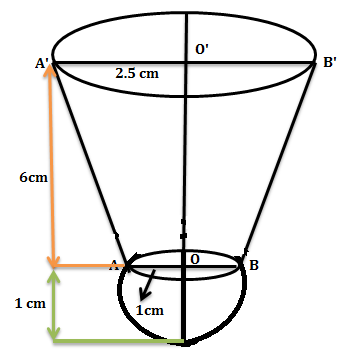QUESTION

# A shuttlecock used for playing badminton has the shape of a frustum of a cone mounted on a hemisphere as shown in the figure. The external diameters of the frustum are 5cm and 2cm, the height of the entire shuttlecock is 7cm. Find its external surface area.Hint: For this question, you can use the formula of slant height of the cone and the external surface area of the shuttlecock is the sum of the curved surface area of the frustum and surface area of hemisphere.

Let ${r_1}$ be the radius of the lower end of the frustum and ${r_2}$ be the radius of the upper end of the frustum. Let $h$ and $l$ be the height and slant height of the frustum respectively.

Given that, ${r_1} = 1{\text{ cm, }}{r_2} = 2.5{\text{ cm, }}h = 6{\text{ cm}}$ and then the slant height of the frustum is given by

$l = \sqrt {{h^2} + {{\left( {{r_1} - {r_2}} \right)}^2}} ................(1)$

Now put all the values of ${r_1},{r_2},h$ in the equation (1), we get

$l = \sqrt {36 + {{\left( {2.5 - 1} \right)}^2}}$

$l = \sqrt {36 + {{\left( {1.5} \right)}^2}}$

$l = \sqrt {36 + 2.25}$

$l = \sqrt {38.25}$

$l = 6.18$

Hence the slant height of the frustum is 6 cm.

We know that,

External surface area of shuttlecock = Curved surface area of the frustum + Surface area of the hemisphere

External surface area of shuttlecock $= \pi \left( {{r_1} + {r_2}} \right)l + 2\pi {r_1}^2..............(2)$

Now put all the values ${r_1},{r_2},l$ in the equation (2), we get

External surface area of shuttlecock $= \left\{ {\pi \left( {1 + 2.5} \right) \times 6.18 + 2 \times \pi \times {{(1)}^2}} \right\}.........(3)$

Also put the value of $\pi = 3.14$ in the equation (3), we get

External surface area of shuttlecock $= \left\{ {3.14 \times 3.5 \times 6.18 + 2 \times 3.14} \right\}$

External surface area of shuttlecock $= \left\{ {67.92 + 6.28} \right\}$

External surface area of shuttlecock $= 74.26$

Hence the external surface area of the shuttlecock is 74.26 ${cm^2}$.

Note: The possibility for the mistake is that you might get confused with the concept that the shape of the shuttlecock is equal to the sum of frustum of a cone and a hemi sphere, not a cone and a sphere.